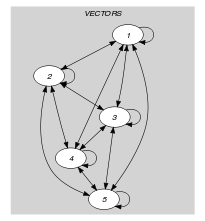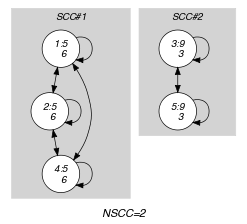## 5.42. atmost_nvector

Origin
Constraint

$\mathrm{𝚊𝚝𝚖𝚘𝚜𝚝}_\mathrm{𝚗𝚟𝚎𝚌𝚝𝚘𝚛}\left(\mathrm{𝙽𝚅𝙴𝙲},\mathrm{𝚅𝙴𝙲𝚃𝙾𝚁𝚂}\right)$

Type
 $\mathrm{𝚅𝙴𝙲𝚃𝙾𝚁}$ $\mathrm{𝚌𝚘𝚕𝚕𝚎𝚌𝚝𝚒𝚘𝚗}\left(\mathrm{𝚟𝚊𝚛}-\mathrm{𝚍𝚟𝚊𝚛}\right)$
Arguments
 $\mathrm{𝙽𝚅𝙴𝙲}$ $\mathrm{𝚍𝚟𝚊𝚛}$ $\mathrm{𝚅𝙴𝙲𝚃𝙾𝚁𝚂}$ $\mathrm{𝚌𝚘𝚕𝚕𝚎𝚌𝚝𝚒𝚘𝚗}\left(\mathrm{𝚟𝚎𝚌}-\mathrm{𝚅𝙴𝙲𝚃𝙾𝚁}\right)$
Restrictions
 $|\mathrm{𝚅𝙴𝙲𝚃𝙾𝚁}|\ge 1$ $\mathrm{𝙽𝚅𝙴𝙲}\ge \mathrm{𝚖𝚒𝚗}\left(1,|\mathrm{𝚅𝙴𝙲𝚃𝙾𝚁𝚂}|\right)$ $\mathrm{𝚛𝚎𝚚𝚞𝚒𝚛𝚎𝚍}$$\left(\mathrm{𝚅𝙴𝙲𝚃𝙾𝚁𝚂},\mathrm{𝚟𝚎𝚌}\right)$ $\mathrm{𝚜𝚊𝚖𝚎}_\mathrm{𝚜𝚒𝚣𝚎}$$\left(\mathrm{𝚅𝙴𝙲𝚃𝙾𝚁𝚂},\mathrm{𝚟𝚎𝚌}\right)$
Purpose

The number of distinct tuples of values taken by the vectors of the collection $\mathrm{𝚅𝙴𝙲𝚃𝙾𝚁𝚂}$ is less than or equal to $\mathrm{𝙽𝚅𝙴𝙲}$. Two tuples of values $〈{A}_{1},{A}_{2},\cdots ,{A}_{m}〉$ and $〈{B}_{1},{B}_{2},\cdots ,{B}_{m}〉$ are $distinct$ if and only if there exist an integer $i\in \left[1,m\right]$ such that ${A}_{i}\ne {B}_{i}$.

Example
$\left(\begin{array}{c}3,〈\begin{array}{c}\mathrm{𝚟𝚎𝚌}-〈5,6〉,\hfill \\ \mathrm{𝚟𝚎𝚌}-〈5,6〉,\hfill \\ \mathrm{𝚟𝚎𝚌}-〈9,3〉,\hfill \\ \mathrm{𝚟𝚎𝚌}-〈5,6〉,\hfill \\ \mathrm{𝚟𝚎𝚌}-〈9,3〉\hfill \end{array}〉\hfill \end{array}\right)$

The $\mathrm{𝚊𝚝𝚖𝚘𝚜𝚝}_\mathrm{𝚗𝚟𝚎𝚌𝚝𝚘𝚛}$ constraint holds since the collection $\mathrm{𝚅𝙴𝙲𝚃𝙾𝚁𝚂}$ involves at most 3 distinct tuples of values (i.e., in fact the 2 distinct tuples $〈5,6〉$ and $〈9,3〉$).

Typical
 $|\mathrm{𝚅𝙴𝙲𝚃𝙾𝚁}|>1$ $\mathrm{𝙽𝚅𝙴𝙲}>1$ $\mathrm{𝙽𝚅𝙴𝙲}<|\mathrm{𝚅𝙴𝙲𝚃𝙾𝚁𝚂}|$ $|\mathrm{𝚅𝙴𝙲𝚃𝙾𝚁𝚂}|>1$
Symmetries
• $\mathrm{𝙽𝚅𝙴𝙲}$ can be increased.

• Items of $\mathrm{𝚅𝙴𝙲𝚃𝙾𝚁𝚂}$ are permutable.

• Items of $\mathrm{𝚅𝙴𝙲𝚃𝙾𝚁𝚂}.\mathrm{𝚟𝚎𝚌}$ are permutable (same permutation used).

• All occurrences of two distinct tuples of values of $\mathrm{𝚅𝙴𝙲𝚃𝙾𝚁𝚂}.\mathrm{𝚟𝚎𝚌}$ can be swapped; all occurrences of a tuple of values of $\mathrm{𝚅𝙴𝙲𝚃𝙾𝚁𝚂}.\mathrm{𝚟𝚎𝚌}$ can be renamed to any unused tuple of values.

Arg. properties

Contractible wrt. $\mathrm{𝚅𝙴𝙲𝚃𝙾𝚁𝚂}$.

Reformulation

By introducing an extra variable $\mathrm{𝙽𝚅}\in \left[0,|\mathrm{𝚅𝙴𝙲𝚃𝙾𝚁𝚂}|\right]$, the $\mathrm{𝚊𝚝𝚖𝚘𝚜𝚝}_\mathrm{𝚗𝚟𝚎𝚌𝚝𝚘𝚛}$$\left(\mathrm{𝙽𝚅},\mathrm{𝚅𝙴𝙲𝚃𝙾𝚁𝚂}\right)$ constraint can be expressed in term of an $\mathrm{𝚗𝚟𝚎𝚌𝚝𝚘𝚛}$$\left(\mathrm{𝙽𝚅},\mathrm{𝚅𝙴𝙲𝚃𝙾𝚁𝚂}\right)$ constraint and of an inequality constraint $\mathrm{𝙽𝚅}\le \mathrm{𝙽𝚅𝙴𝙲}$.

implied by: $\mathrm{𝚗𝚟𝚎𝚌𝚝𝚘𝚛}$ ($\le$ $\mathrm{𝙽𝚅𝙴𝙲}$ replaced by $=$ $\mathrm{𝙽𝚅𝙴𝙲}$), $\mathrm{𝚘𝚛𝚍𝚎𝚛𝚎𝚍}_\mathrm{𝚊𝚝𝚖𝚘𝚜𝚝}_\mathrm{𝚗𝚟𝚎𝚌𝚝𝚘𝚛}$.

Keywords
Arc input(s)

$\mathrm{𝚅𝙴𝙲𝚃𝙾𝚁𝚂}$

Arc generator
$\mathrm{𝐶𝐿𝐼𝑄𝑈𝐸}$$↦\mathrm{𝚌𝚘𝚕𝚕𝚎𝚌𝚝𝚒𝚘𝚗}\left(\mathrm{𝚟𝚎𝚌𝚝𝚘𝚛𝚜}\mathtt{1},\mathrm{𝚟𝚎𝚌𝚝𝚘𝚛𝚜}\mathtt{2}\right)$

Arc arity
Arc constraint(s)
$\mathrm{𝚕𝚎𝚡}_\mathrm{𝚎𝚚𝚞𝚊𝚕}$$\left(\mathrm{𝚟𝚎𝚌𝚝𝚘𝚛𝚜}\mathtt{1}.\mathrm{𝚟𝚎𝚌},\mathrm{𝚟𝚎𝚌𝚝𝚘𝚛𝚜}\mathtt{2}.\mathrm{𝚟𝚎𝚌}\right)$
Graph property(ies)
$\mathrm{𝐍𝐒𝐂𝐂}$$\le \mathrm{𝙽𝚅𝙴𝙲}$

Graph class
$\mathrm{𝙴𝚀𝚄𝙸𝚅𝙰𝙻𝙴𝙽𝙲𝙴}$

Graph model

Parts (A) and (B) of Figure 5.42.1 respectively show the initial and final graph associated with the Example slot. Since we use the $\mathrm{𝐍𝐒𝐂𝐂}$ graph property we show the different strongly connected components of the final graph. Each strongly connected component corresponds to a tuple of values that is assigned to some vectors of the $\mathrm{𝚅𝙴𝙲𝚃𝙾𝚁𝚂}$ collection. The 2 following tuple of values $〈5,6〉$ and $〈9,3〉$ are used by the vectors of the $\mathrm{𝚅𝙴𝙲𝚃𝙾𝚁𝚂}$ collection.

##### Figure 5.42.1. Initial and final graph of the $\mathrm{𝚊𝚝𝚖𝚘𝚜𝚝}_\mathrm{𝚗𝚟𝚎𝚌𝚝𝚘𝚛}$ constraint(a) (b)